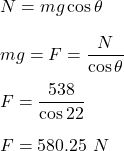## A box at rest on a ramp at an incline of 22°. The normal force on the box is 538 N

Question

A box at rest on a ramp at an incline of 22°. The normal force on the box is 538 N

in progress 0
3 months 2021-08-06T09:48:07+00:00 1 Answers 62 views 0

F = 580.25 N

Explanation:

It is given that,

A box at rest on a ramp at an incline of 22°

The normal force on the box is 538 N

We need to find the gravitational force on the box.

The force of gravity acting on an object is equal to its weight. So,

F = mg

But here the box is incline at an angle of 22 degrees

It means that mg will resolve in rectangular components such that, normal force is :So, the gravitational force on the box is 580.25 N.